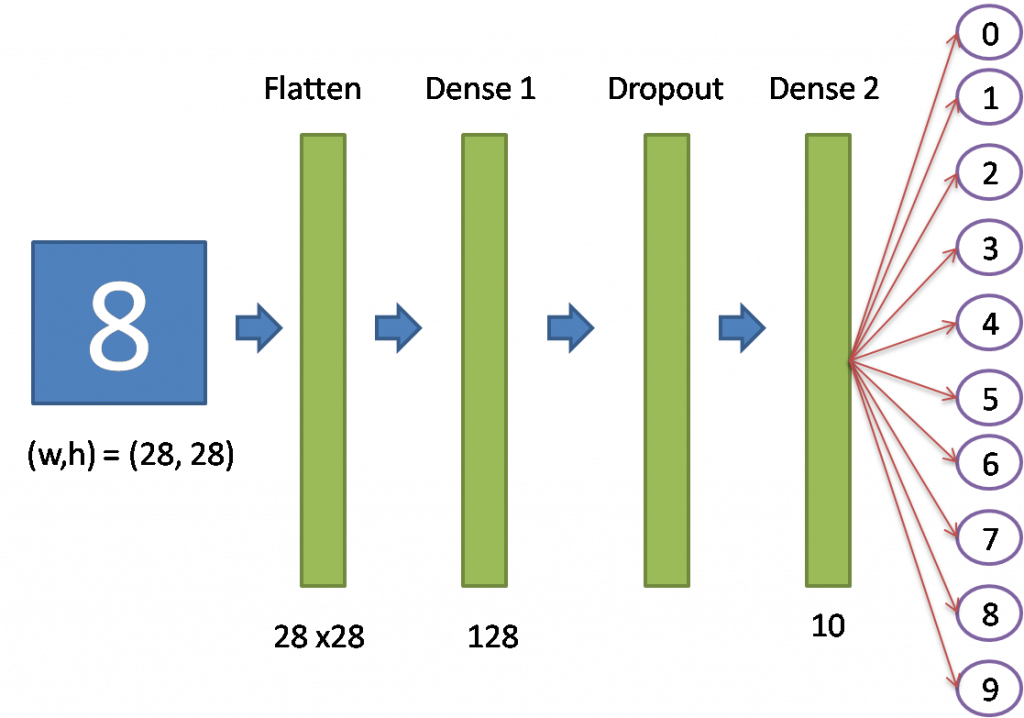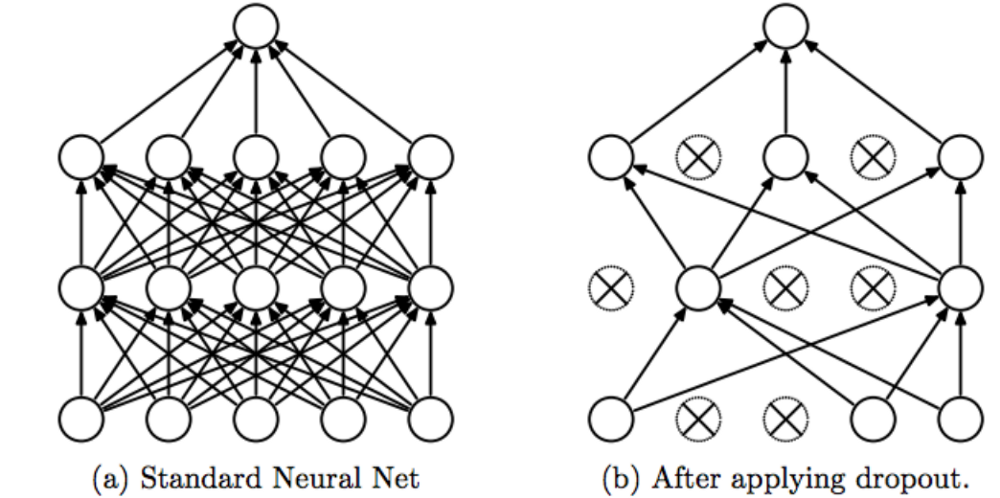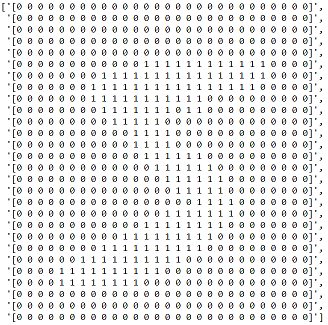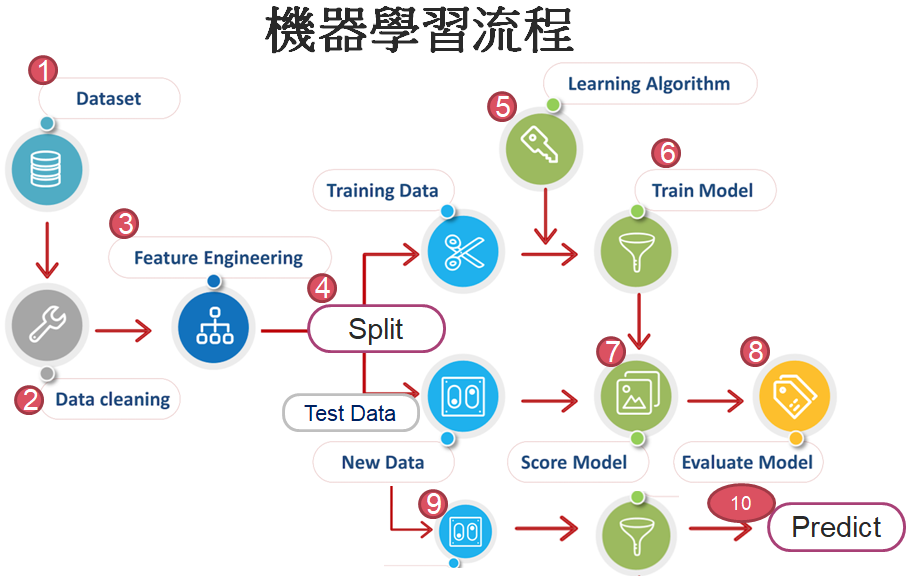#DAY 3
2
AI & Data

## 前言

2017年我使用 Keras 獨立套件撰寫辨識手寫阿拉伯數字的程式，請參閱【Day 02：撰寫第一支 Neural Network 程式 -- 阿拉伯數字辨識】，現在，我們改用TensorFlow Keras 寫類似的程式，讀者可比較其差異，其實，大部份的程式是相同的，只是命名空間不同罷了。

## Tensorflow 的反攻

Tensorflow 1.x 版使用會話(Session)及運算圖(Computational Grahp)的概念，撰寫一支簡單的程式就要花費好大的功夫，被 Facebook PyTorch 批評的體無完膚，包括：

1. 程式觀念複雜難懂：Tensorflow把簡單的事情複雜化，Keras 則把複雜的事情過於簡化。
2. 除錯不易：須等到運算圖(Computational Grahp)執行完畢，才可以查看過程中的變數值。
3. 與 Python 整合度差，無法在模型中直接使用 Python 的 if/else。

1. 把運算圖(Computational Grahp)隱藏起來，預設模式改為 Eager Mode，隨時可列印(print)變數值。
3. 重新開發 Keras，並與其他模組整合。

``````import tensorflow as tf
mnist = tf.keras.datasets.mnist

# 匯入 MNIST 手寫阿拉伯數字 訓練資料
# 特徵縮放，使用常態化(Normalization)，公式 = (x - min) / (max - min)
# 顏色範圍：0~255，所以，公式簡化為 x / 255
x_train, x_test = x_train / 255.0, x_test / 255.0

# 建立模型
model = tf.keras.models.Sequential([
tf.keras.layers.Flatten(input_shape=(28, 28)),
tf.keras.layers.Dense(128, activation='relu'),
tf.keras.layers.Dropout(0.2),
tf.keras.layers.Dense(10, activation='softmax')
])

# 設定優化器(optimizer)、損失函數(loss)、效能衡量指標(metrics)的類別
loss='sparse_categorical_crossentropy',
metrics=['accuracy'])

# 模型訓練
model.fit(x_train, y_train, epochs=5)
# 模型評估，打分數
model.evaluate(x_test, y_test)
``````1. Flatten Layer：將寬、高各28個像素的圖轉成一維陣列 28 x 28 = 784個特徵。
2. Dense Layer：input為784個特徵，output 為128個神經元，即128條迴歸線。
3. Dropout Layer：在訓練週期隨機丟棄20%的神經元，藉以矯正過度擬合的現象。4. 最後一個 Dense Layer：輸出10個神經元，透過 softmax activation function，轉成機率，即0~9的預測機率，選擇最大機率者為預測值。

## 強化

1. 顯示圖片資料。
``````# 將非0的數字轉為1，顯示第1張圖片
data = x_train.copy()
data[data>0]=1

# 將轉換後二維內容顯示出來，隱約可以看出數字為 5
text_image=[]
for i in range(data.shape):
text_image.append(''.join(str(data[i])))
text_image
``````1. 訓練資料切成兩份，驗證資料占兩成。
``````# 訓練
history = model.fit(x_train_norm, y_train, epochs=5, validation_split=0.2)
``````
1. 對訓練過程的準確度/損失函數繪圖。
``````# 對訓練過程的準確度繪圖
import matplotlib.pyplot as plt
plt.plot(history.history['accuracy'], 'r')
plt.plot(history.history['val_accuracy'], 'g')

# 對訓練過程的損失函數繪圖
plt.plot(history.history['loss'], 'r')
plt.plot(history.history['val_loss'], 'g')
``````
1. 實際預測 N 筆，觀察結果，發現第9筆錯誤，顯示錯誤的圖片。
``````# 實際預測 20 筆
predictions = model.predict_classes(x_test_norm)
# get prediction result
print('prediction:', predictions[0:20])
print('actual    :', y_test[0:20])

# 顯示錯誤的資料圖像
X2 = x_test[8,:,:]
plt.imshow(X2.reshape(28,28))
plt.show()
``````1. 使用小畫家，寫0~9，實際測試看看，注意，顏色0為白色，與RGB顏色不同，(0,0,0) 為黑色。
``````# 使用小畫家，寫0~9，實際測試看看
from skimage import io
from skimage.transform import resize
import numpy as np

image_resized = resize(image1, (28, 28), anti_aliasing=True)
X1 = image_resized.reshape(1,28, 28) #/ 255
# 反轉顏色
# 顏色0為白色，與RGB顏色不同，(0,0,0) 為黑色。
X1 = np.abs(1-X1)
predictions = model.predict_classes(X1)
print(predictions)
``````
1. 其他功能：顯示模型的彙總資訊、模型存檔/載入、模型繪圖。
``````# 顯示模型的彙總資訊
model.summary()

# 模型存檔
model.save('model.h5')

# 模型載入

# 繪製模型
# 將安裝路徑 C:\Program Files (x86)\Graphviz2.38\bin 新增至環境變數 path 中
# pip install graphviz
# pip install pydotplus
tf.keras.utils.plot_model(model, to_file='model.png')
``````

## 結論

1. 神經網路並不是真的認識圖片中的數字，它只是將圖片中的像素當作特徵，將訓練資料作歸納，找出0~9的區別。
2. 機器學習並不是規則引擎(Rule Engine)，而是演算法藉由資料，歸納出模型或公式。
3. 機器學習的標準處理流程如下圖，後續再詳加說明。1. 為什麼模型要設成兩層完全連接層(Dense)?
2. 為什麼第一層完全連接層(Dense)要設為 128?
3. activation function、優化器(optimizer)、損失函數(loss)、效能衡量指標(metrics) 要如何選擇?
4. 為什麼使用小畫家，寫0~9實際測試，準確率好像不如測試資料那麼高? 我寫9，永遠辨識錯誤，唉 !
5. 使用像素辨識，好像不是很高明，應該用輪廓或線條比較好吧。
6. 如果要辨識多個數字，例如車牌，要怎麼作?
7. 如果要辨識其他事物，怎麼作?